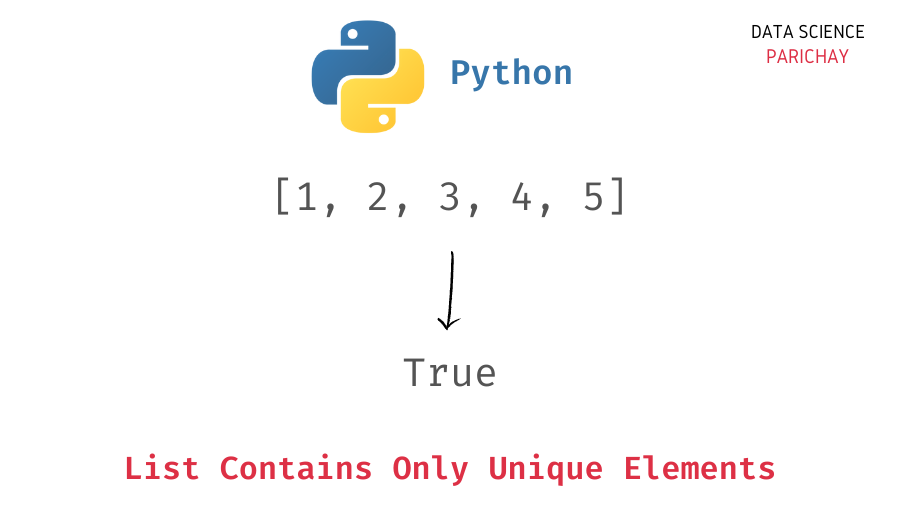# Python – Check If All Elements in a List are Unique

In this tutorial, we will look at how to check if all elements in a list are unique or not in Python with the help of some examples.

You can use the following two methods to check if all elements in a list are unique in Python –

1. Using a set – Compare the length of the list to the length of the set resulting from the list.
2. Checking for any duplicates elments in a list using a loop and another list.

Let’s take a look at both methods in detail with the help of some examples.

Sets are an unordered collection of objects that store only unique elements. In Python, you can compare the length of the list and the length of the set resulting from the list to check if all the elements in the list are unique.

If the length of the list is the same as the length of the set resulting from the list, you can say that the list contains only unique elements.

Here’s an example.

```# create a list
ls = [1, 2, 3, 4]
# check if list contains only unique elements
print(len(ls)==len(set(ls)))```

Output:

`True`

We get `True` as the output because the list `ls` contains only unique elements.

📚 Data Science Programs By Skill Level

Introductory

Intermediate ⭐⭐⭐

🔎 Find Data Science Programs 👨‍💻 111,889 already enrolled

Disclaimer: Data Science Parichay is reader supported. When you purchase a course through a link on this site, we may earn a small commission at no additional cost to you. Earned commissions help support this website and its team of writers.

Let’s look at another example.

```# create a list
ls = [1, 2, 3, 4, 3, 5]
# check if list contains only unique elements
print(len(ls)==len(set(ls)))```

Output:

`False`

Here we get `False` as the output because the list `ls` does not contain only unique values. The value `3` occurs in the list twice.

In this method, the idea is to iterate through the values in the list and check for duplicates in the list. If the list contains any duplicates, it does not have only unique values.

Let’s take a look at an example.

```# function to check if list contains only unique elements
def check_ls_unique(ls):
encountered_ls = []
for item in ls:
if item in encountered_ls:
return False
encountered_ls.append(item)
return True

# create a list
ls = [1, 2, 3, 4]
# check if ls contains only unique values
print(check_ls_unique(ls))```

Output:

`True`

We get `True` as the output because the list `ls` contains only unique elements.

Let’s take a look at another example.

```# function to check if list contains only unique elements
def check_ls_unique(ls):
encountered_ls = []
for item in ls:
if item in encountered_ls:
return False
encountered_ls.append(item)
return True

# create a list
ls = [1, 2, 3, 4, 3, 5]
# check if ls contains only unique values
print(check_ls_unique(ls))```

Output:

`False`

Here we get `False` as the output because the element `3` occurs in the list more than once.

You might also be interested in –

•